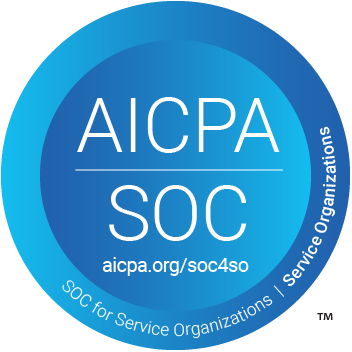# How to Calculate the Discount Rate Implicit in the Lease

by Lucas Russell | 2021-04-27

## What is the Implicit rate in the lease?

There will be more focus on the implicit rate in the lease due to the adoption of ASC 842. Under the new lease accounting standard, all leases are recognized on the balance sheet with a right of use asset and a lease liability regardless of the lease classification. The lease liability is the present value of all known future lease payments at a certain point in time, with a few exceptions. To calculate the present value of the lease liability, there are three key inputs:

• lease payments: the cash outflow amount which is stated within the lease agreement
• lease term: the cash outflow frequency, which is stated within the lease agreement
• discount rate: input to present value the cash outflows, not stated within the lease agreement

For information on how to calculate the present value of lease payments, refer here.

So what do these three inputs have to do with the implicit rate in the lease? As per ASC 842 it states:

For a lessee, the discount rate for the lease is the rate implicit in the lease unless that rate cannot be readily determined. In that case, the lessee is required to use its incremental borrowing rate.

For a lessor, the discount rate for the lease is the rate implicit in the lease.

Regardless of the party in the lease transaction, the implicit rate in the lease is an important figure to calculate!

#### Discount Rate and the Implicit Rate in the Lease

As noted above, to calculate the present value of the lease liability, a discount rate is required. Unless the discount rate is equal to the current inflation rate, it is assumed there is an interest portion to these cash outflows.

In other words, the discount rate is the interest rate being charged by the lessor to the lessee for leasing the asset. That's why from a lessee's perspective, lease payments consist of a portion of principal and interest [ 1 ], unlike other commercial transactions like a loan where the interest rate is clearly stated and a critical input in deciding if you'll enter into that transaction or not. With a lease agreement, a lessee will unlikely be communicated the interest rate in the lease payments.

That's because, and as we go through the inputs required to calculate the rate implicit in the lease, it will become more apparent, the lessee is not privy to this information. Why? Because of commercial sensitivity for the lessor's perspective, not many companies disclosure to their customers how much profit they're exactly making off them ;). A clue of the difficulty of acquiring this number is in its name. It's the implicit rate in the lease, not the explicit rate.

Conversely, for a lessor who has determined the commercial terms of the lease and owns the leased asset, the implicit rate in the lease is far easier calculate.

Despite these challenges, the new lease accounting standard does prescribe the lessee to use the rate implicit in the lease if available as the discount rate.

[ 1 ] For operating lease payments under ASC 842, the interest expense incurred on the lease liability is classified as a "lease expense" as opposed to an "interest expense". If you're curious about the differences between an operating and finance lease under ASC 842, refer here.

## Key inputs and definitions

There are several inputs required to calculate the rate implicit in the lease. But before going any further, we should probably define these terms are starting with the implicit rate in the lease!

ASC 842 defines the implicit rate of the lease as:

The rate of interest that, at a given date, causes the aggregate present value of (a) the lease payments and (b) the amount that a lessor expects to derive from the underlying asset following the end of the lease term to equal the sum of (1) the fair value of the underlying asset minus any related investment tax credit retained and expected to be realized by the lessor and (2) any deferred initial direct costs of the lessor.

So what does that mean? Here is the above definition summarized and each key term defined:Defined terms:

• Present value: Is the current value of a future sum of money or stream of cash flow given a specified rate of return. For further details on the definition of present value, refer here
• Lease payments: The known payments at the outset of the contact between the lessee and lessor
• Residual asset value: the estimated value of the leased asset at the end of the agreement's term that is not the responsibility of the lessee.
• Fair value: The price that would be received to sell an asset or paid to transfer a liability in an orderly transaction between market participants at the measurement date.
• Investment tax credit: This amount will be deducted from the fair value amount of the asset
• Deferred lessor initial recognition costs: Incremental costs of a lease that would not have been incurred if the lease had not been obtained. These amounts are added to the fair value.

With each input broken down and defined for calculating the lease's implicit interest rate, we're ready for an example.

## Example 1

Let’s note the following inputs:

• Fair value of the asset: (1) \$50,000
• Deferred lessor costs: (2) \$5,000
• Lease payments: (a) 8 payments starting January 2021
• Payment frequency: Annually
• P/V Unguaranteed residual value: (b) \$55,000

To put the above in a table it will look like this:From the above inputs, you can determine the rate implicit in the lease by using Microsoft Excel's IRR function. In this example, the rate implicit in the lease is 4.58%.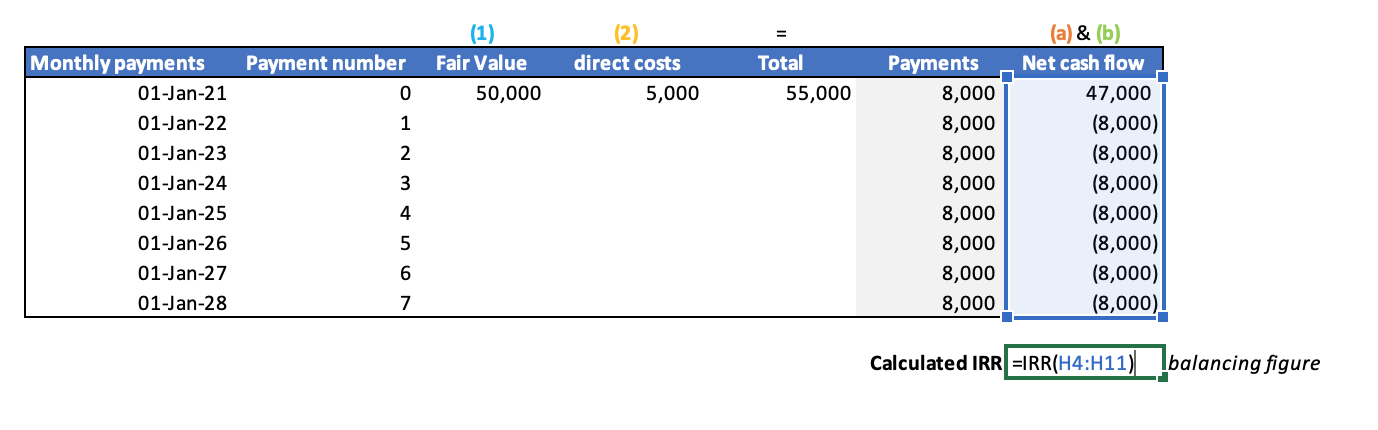Before going further we’ll break down what the IRR function does in Excel:

Description

Returns the internal rate of return for a series of cash flows represented by the numbers in values. These cash flows do not have to be even, as they would be for an annuity. However, the cash flows must occur at regular intervals, such as monthly or annually. The internal rate of return is the interest rate received for an investment consisting of payments (negative values) and income (positive values) that occur at regular periods.

Syntax

IRR(values, [guess])

The IRR function syntax has the following arguments:

> Values Required. An array or a reference to cells that contain numbers for which you want to calculate the internal rate of return.

• values must contain at least one positive value and one negative value to calculate the internal rate of return.
• IRR uses the order of values to interpret the order of cash flows. Be sure to enter your payment and income values in the sequence you want.
• If an array or reference argument contains text, logical values, or empty cells, those values are ignored.

> Guess (Optional.) A number that you guess is close to the result of IRR.

• Microsoft Excel uses an iterative technique for calculating IRR. Starting with guess, IRR cycles through the calculation until the result is accurate within 0.00001 percent. If IRR can't find a result that works after 20 tries, the #NUM! error value is returned.
• In most cases you do not need to provide guess for the IRR calculation. If guess is omitted, it is assumed to be 0.1 (10 percent).
• If IRR gives the #NUM! error value, or if the result is not close to what you expected, try again with a different value for guess.

IRR is closely related to NPV, the net present value function. The rate of return calculated by IRR is the interest rate corresponding to a 0 (zero) net present value.

## Recalculating the implicit rate of the lease

Based on the inputs in Example 1, the calculated implicit rate in the lease is 4.58%. Applying 4.58% as the discount rate, the present value of the future lease payments should equate to \$55,000. This can be demonstrated in Excel using either PV or NPV function. Firstly here's how to achieve it using the PV function within Excel: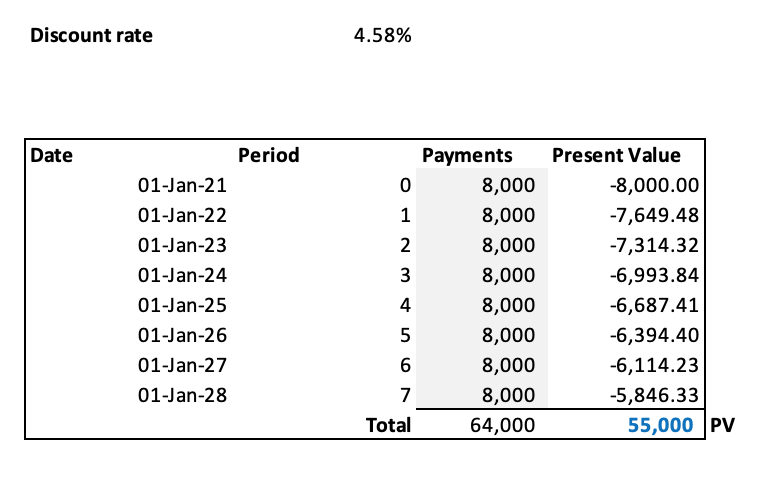The formula input to calculate the present value of each payment is: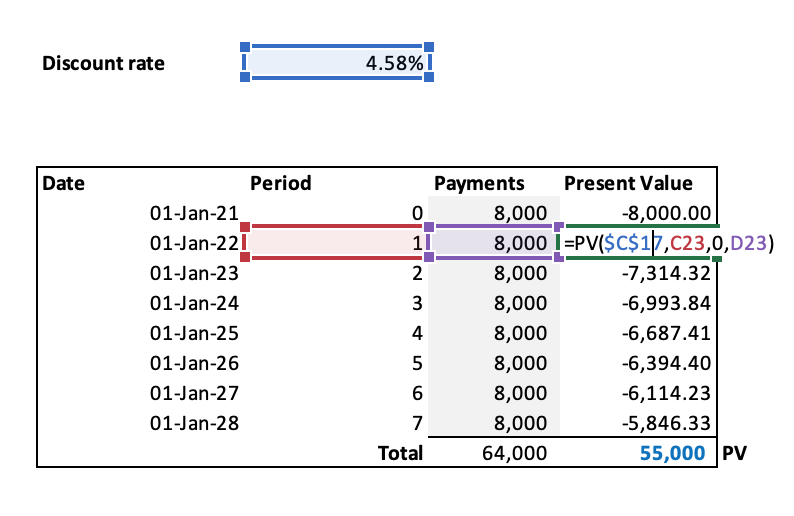Request our free present value tool at [email protected] to quickly recreate the present value calculations.

## An imperfect way to calculate the implicit rate in the lease

To calculate the present value of \$55,000 is not exactly straightforward, this is highlighted when using the NPV function in excel: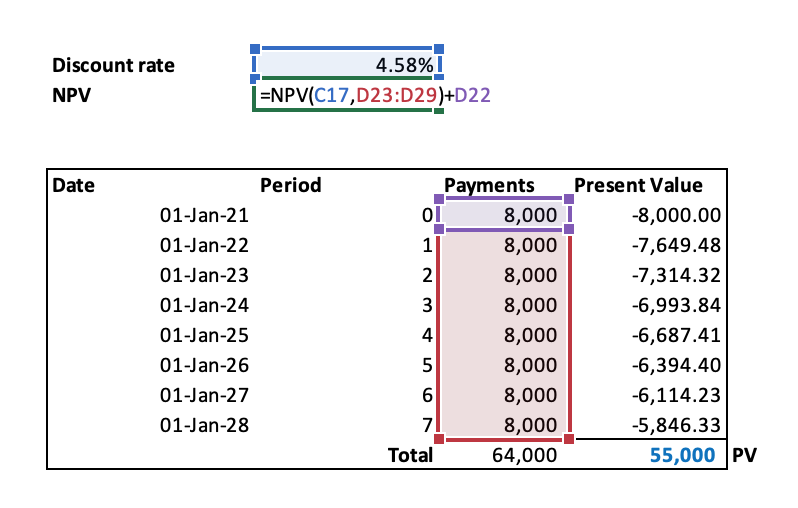Using those above inputs will result in the NPV of \$55k: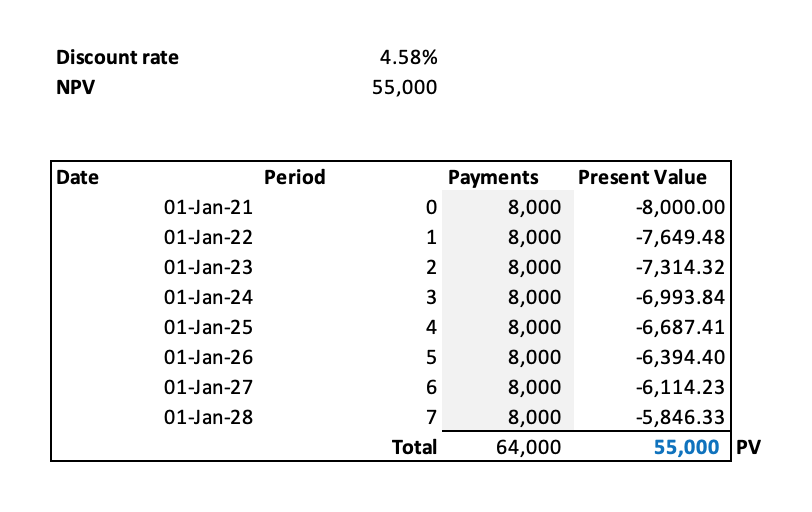You will see it’s necessary to add the payment of \$8,000 occurring on January 1, 2021, with the amount not included in the NPV formula. Why?

The IRR, PV & NPV formulas within Excel assume the cash flows must occur at regular intervals, such as monthly or annually. If you were to include the \$8,000 payment occurring on January 1, Excel will assume it's the first value to be present valued, both PV and NPV formulas do not consider the date of the payment. In this case, because the payment is on day 1 of the lease commencement technically it doesn't need to be present valued anyway. The point is to highlight calculating the implicit rate of the lease using the IRR Excel functional is imperfect as it assumes all payments occur at regular intervals. In the world of lease accounting, all payments do not occur at regular intervals.

Using the XNPV function within Excel can clearly highlight the difference in how different payment schedules can impact the present value of the lease payments. This is because the XNPV function takes into consideration the date of the payment.

XNPV formula of lease payments received monthly: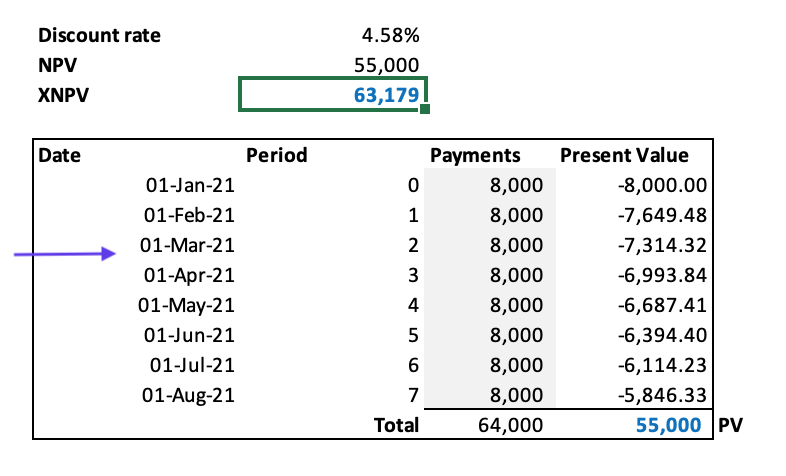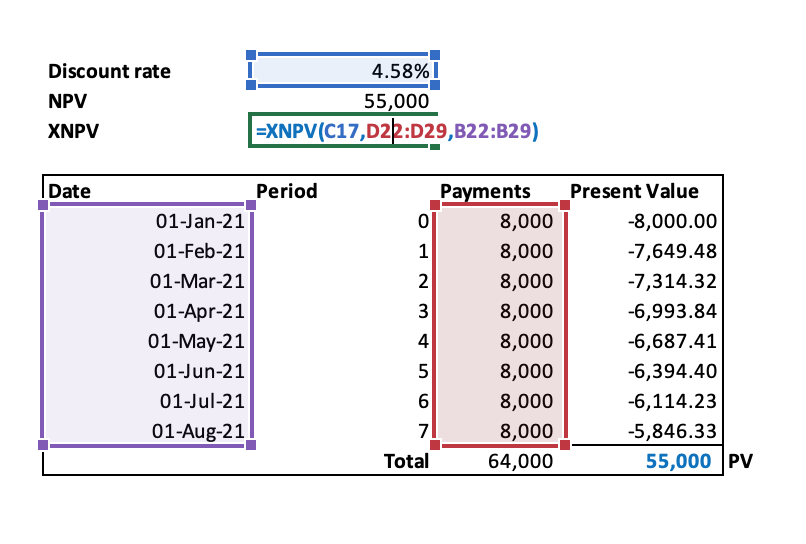XNPV formula of lease payments received annually: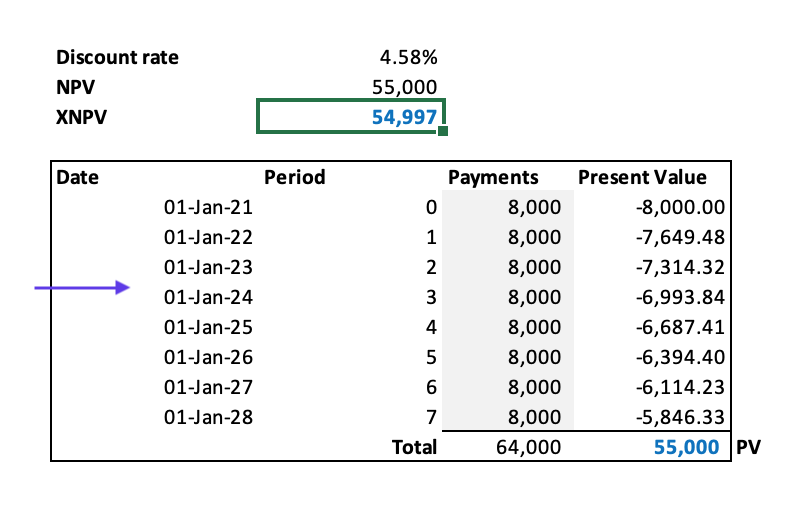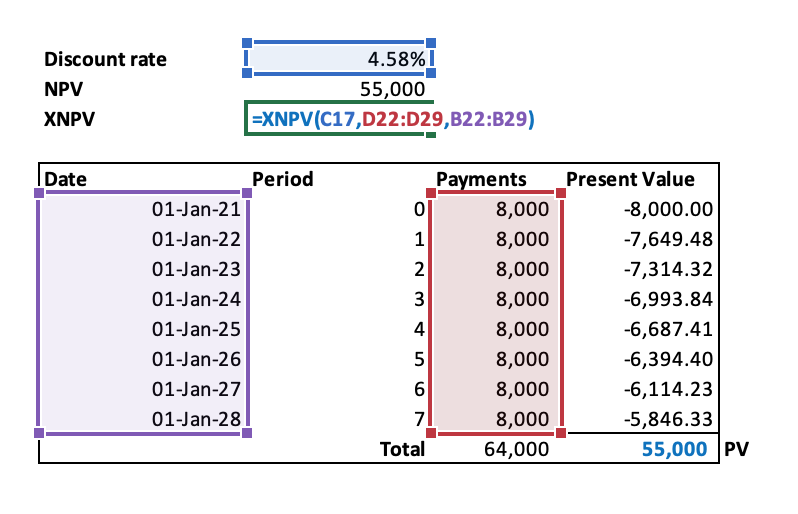An \$8,000 difference is the result, while the IRR, NPV & PV functions do not even pick up that difference. For more details on different ways to present value lease payments refer here.

## Conclusion

This article has provided a solution on how to calculate the implicit rate in the lease. However, the accounting standards do not get into the nuances of present value techniques. As demonstrated above, each Excel present value function can result in differing numbers. Despite this, using the IRR function within Excel, once you have access to all the necessary inputs of the implicit rate, is a simple way to calculate the implicit rate in the lease.

## Lease accounting is time-consuming and material

The complexities with ASC 842 compliance are numerous, ranging from determining the discount rate of a lease to modification accounting. So why not use Cradle to remove all unnecessary tasks associated with the ASC 842 while at the same time implementing efficiencies and improving accuracy?

Book demo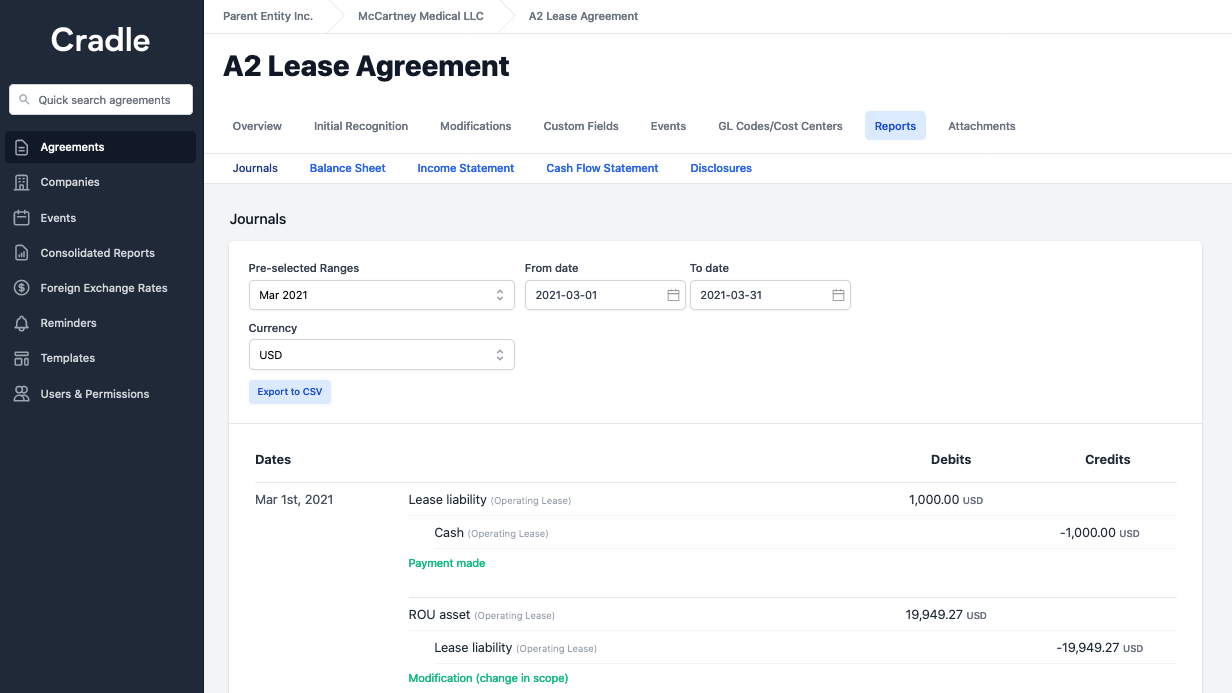## FooterHere at Cradle, our mission is simple; it's at the foundation of everything that we do. We want to make accountants' lives easier by leveraging technology to free up their time to focus on running the business.

140 Yonge St.
Suite 200
Toronto, ON M5C 1X6

US